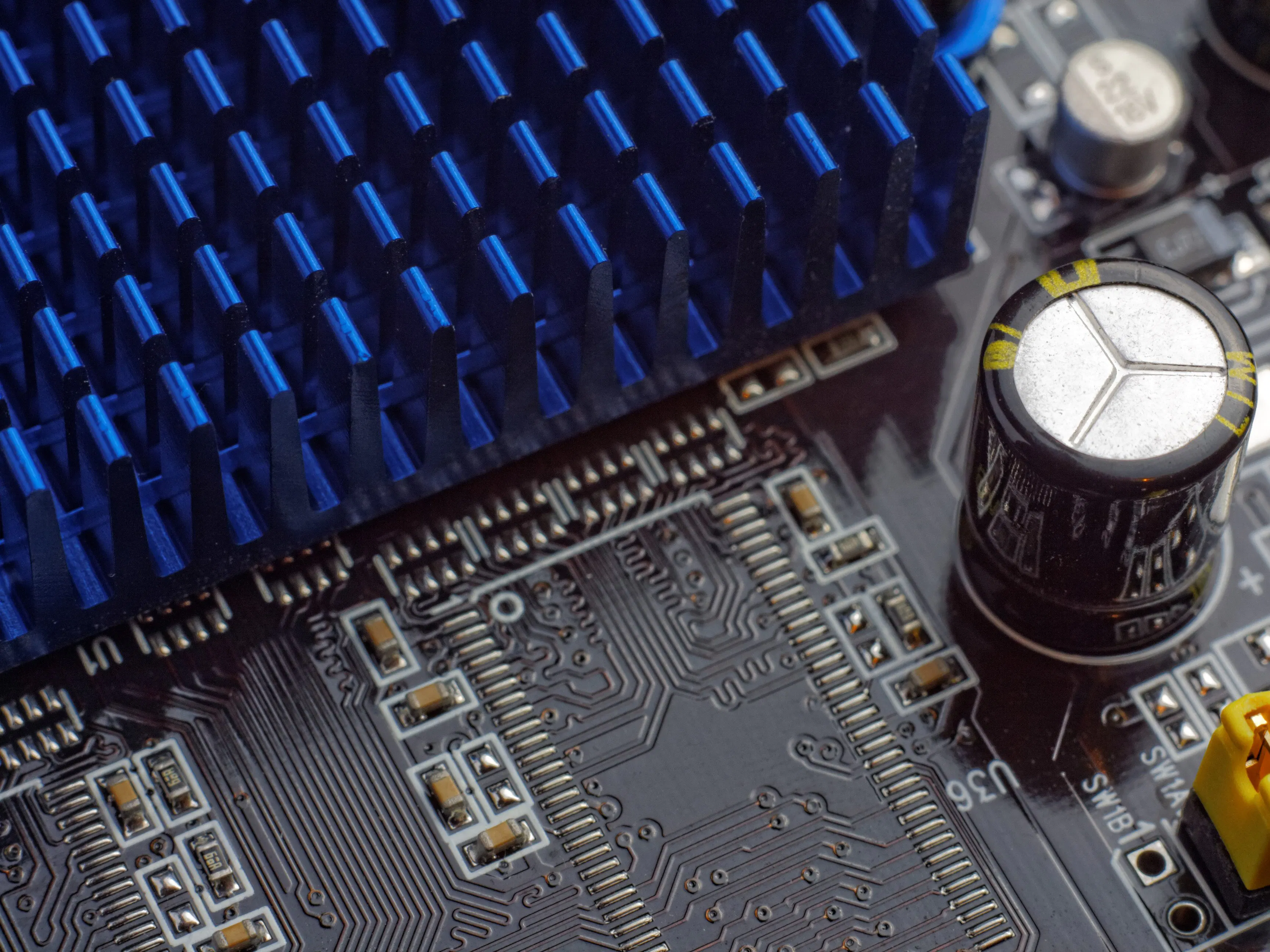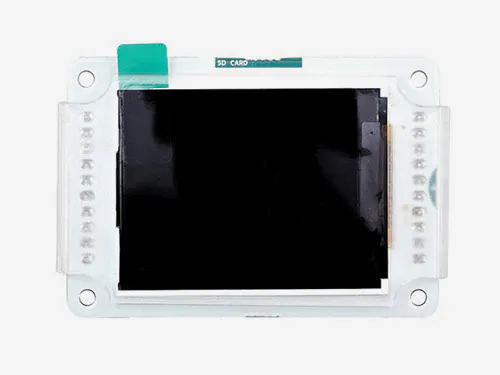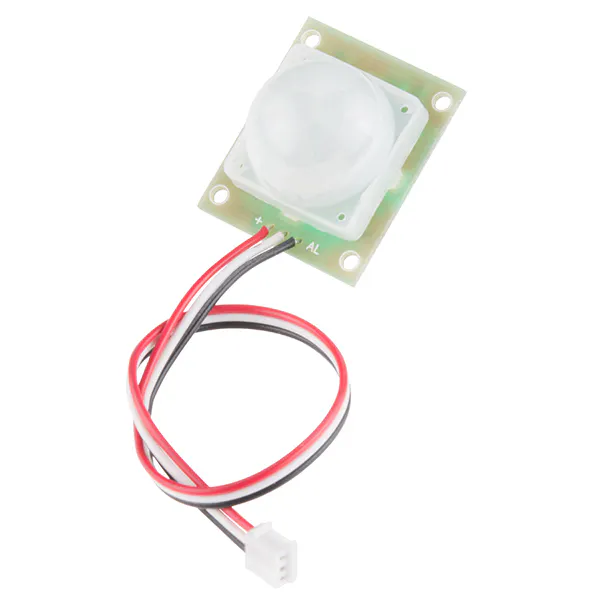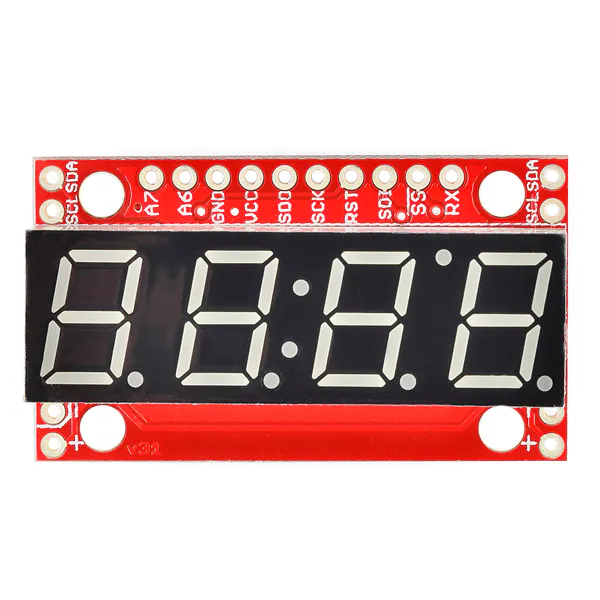# Getting Started with Sainsmart Uno Deluxe Learning kit

Basic starter tutorial with this deluxe kit: showing interfacing and using the sensors and board given in the kit.

BeginnerProtip1 hour1,920## Things used in this project

### Hardware components

 Arduino Uno Deluxe Learning kit (CHIKU) with Relay, Servo, Ultrasonic sensor, LED, Potentiometer
×1Arduino UNO
×1Arduino LCD Screen
×1PIR Motion Sensor (generic)
×1SparkFun 7-Segment Serial Display - Red
×1Jumper wires (generic)
×1

### Software apps and online servicesArduino IDE

### Hand tools and fabrication machinesSoldering iron (generic)

## Code

### Interfacing Piezo Element

C/C++
```int sensoroutput = 4; // the analog pin connected to the sensor
int ledoutput = 0; // pin connected to LED
int THRESHOLD = 100;
void setup()
{
pinMode(ledPin, OUTPUT);   // this function is used to declare led connected pin as output
}
void loop()
{
int value = analogRead(sensoroutput);  // function to read analog voltage from sensor
if (value >= THRESHOLD)                    // function to check voltage level from sensor
{
digitalWrite(ledoutput, HIGH);
delay(100); // to make the LED visible
}
else
digitalWrite(ledoutput, LOW);
}
```

### Interfacing Servo Motor

C/C++
```#include <Servo.h>

Servo myservo;  // create servo object to control a servo
// twelve servo objects can be created on most boards

int pos = 0;    // variable to store the servo position

void setup() {
myservo.attach(9);  // attaches the servo on pin 9 to the servo object
}

void loop() {
for (pos = 0; pos <= 180; pos += 1) { // goes from 0 degrees to 180 degrees
// in steps of 1 degree
myservo.write(pos);              // tell servo to go to position in variable 'pos'
delay(15);                       // waits 15ms for the servo to reach the position
}
for (pos = 180; pos >= 0; pos -= 1) { // goes from 180 degrees to 0 degrees
myservo.write(pos);              // tell servo to go to position in variable 'pos'
delay(15);                       // waits 15ms for the servo to reach the position
}
}
```

### For Interfacing Seven Segment Display

C/C++
```void setup()
{
// define pin modes

pinMode(2,OUTPUT);
pinMode(3,OUTPUT);
pinMode(4,OUTPUT);
pinMode(5,OUTPUT);
pinMode(6,OUTPUT);
pinMode(7,OUTPUT);
pinMode(8,OUTPUT);

}

void loop()
{
// loop to turn leds od seven seg ON

for(int i=2;i<9;i++)
{
digitalWrite(i,HIGH);
delay(600);
}

// loop to turn leds od seven seg OFF
for(int i=2;i<9;i++)
{
digitalWrite(i,LOW);
delay(600);
}

delay(1000);

}
```

### Controlling LED Using IR Remote

C/C++
```/*
Some Sample code of how to use your IR remote

* Lets get started:

The IR sensor's pins are attached to Arduino as so:
Pin 1 to Vout (pin 11 on Arduino)
Pin 2 to GND
Pin 3 to Vcc (+5v from Arduino)

*/

#include <IRremote.h>

int IRpin = 11;  // pin for the IR sensor
int LED = 13;    // LED pin
IRrecv irrecv(IRpin);
decode_results results;

boolean LEDon = true; // initializing LEDon as true

void setup()
{
Serial.begin(9600);
pinMode(LED, OUTPUT);
}

void loop()
{

if (irrecv.decode(&results))
{

irrecv.resume();   // Receive the next value
}

if (results.value == 0)  // change zero to your IR remote button number
{
if (LEDon == true)   // is LEDon equal to true?
{
LEDon = false;
digitalWrite(LED, HIGH);
delay(100);      // keeps the transistion smooth

}

else
{
LEDon = true;
digitalWrite(LED, LOW);
delay(100);

}

}

}
```

### Interfacing Ultrasonic Range Sensor

C/C++
```/*
* Ultrasonic Sensor HC-SR04 interfacing with Arduino.
*/
// defining the pins
const int trigPin = 9;
const int echoPin = 10;
// defining variables
long duration;
int distance;
void setup() {
pinMode(trigPin, OUTPUT); // Sets the trigPin as an Output
pinMode(echoPin, INPUT); // Sets the echoPin as an Input
Serial.begin(9600); // Starts the serial communication
}
void loop() {
// Clears the trigPin
digitalWrite(trigPin, LOW);
delayMicroseconds(2);
// Sets the trigPin on HIGH state for 10 micro seconds
digitalWrite(trigPin, HIGH);
delayMicroseconds(10);
digitalWrite(trigPin, LOW);
// Reads the echoPin, returns the sound wave travel time in microseconds
duration = pulseIn(echoPin, HIGH);
// Calculating the distance
distance= duration*0.034/2;
// Prints the distance on the Serial Monitor
Serial.print("Distance: ");
Serial.println(distance);
}
```

### Interfacing PIR Motion Sensor

C/C++
```int calibrationTime = 30;
long unsigned int lowIn;
long unsigned int pause = 5000;
boolean lockLow = true;
boolean takeLowTime;
int PIRValue = 0;

void setup()
{
Serial.begin(9600);
pinMode(pirPin, INPUT);
}

void loop()
{
PIRSensor();
}

void PIRSensor()
{
{
if(lockLow)
{
PIRValue = 1;
lockLow = false;
Serial.println("Motion detected.");
delay(50);
}
takeLowTime = true;
}

{

if(takeLowTime){lowIn = millis();takeLowTime = false;}
if(!lockLow && millis() - lowIn > pause)
{
PIRValue = 0;
lockLow = true;
Serial.println("Motion ended.");
delay(50);
}
}
}
```

### Interfacing Joystick

C/C++
```int xPin = A1;
int yPin = A0;
int buttonPin = 2;

int xPosition = 0;
int yPosition = 0;
int buttonState = 0;

void setup() {
// initialize serial communications at 9600 bps:
Serial.begin(9600);

pinMode(xPin, INPUT);
pinMode(yPin, INPUT);

//activate pull-up resistor on the push-button pin
pinMode(buttonPin, INPUT_PULLUP);

// For versions prior to Arduino 1.0.1
// pinMode(buttonPin, INPUT);
// digitalWrite(buttonPin, HIGH);

}

void loop() {

Serial.print("X: ");
Serial.print(xPosition);
Serial.print(" | Y: ");
Serial.print(yPosition);
Serial.print(" | Button: ");
Serial.println(buttonState);

}
```

### Interfacing GLCD

C/C++
```#include <glcd.h>
#include "fonts/allFonts.h" // this makes all the distributed fonts
int count = 0;
void setup()
{
GLCD.Init(NON_INVERTED); // initialize the library
GLCD.ClearScreen();
GLCD.SelectFont(System5x7); // select fixed width system font
GLCD.print("hello world"); // print a message
delay(3000);
}
void loop()
{
GLCD.ClearScreen();
GLCD.DrawRect(0, 0, 64, 61, BLACK); // rectangle in left side of screen
// rounded rectangle around text area
GLCD.DrawRoundRect(68, 0, 58, 61, 5, BLACK);
for(int i=0; i < 62; i += 4)
{
// draw lines from upper left down right side of rectangle
GLCD.DrawLine(1,1,63,i, BLACK);
}
GLCD.DrawCircle(32,31,30,BLACK); // circle centered on left side of screen
GLCD.FillRect(92,40,16,16, WHITE); // clear previous spinner position
GLCD.CursorTo(5,5); // locate cursor for printing text
GLCD.PrintNumber(count); // print a number at current cursor position
count = count + 1;
delay(1000);
}
```

### Interfacing Hall Effect Sensor

C/C++
```volatile byte half_revolutions;
unsigned int rpm;
unsigned long timeold;
void setup()
{
Serial.begin(115200);
attachInterrupt(0, magnet_detect, RISING);//Initialize the intterrupt pin (Arduino digital pin 2)
half_revolutions = 0;
rpm = 0;
timeold = 0;
}
void loop()//Measure RPM
{
if (half_revolutions >= 20) {
rpm = 30*1000/(millis() - timeold)*half_revolutions;
timeold = millis();
half_revolutions = 0;
//Serial.println(rpm,DEC);
}
}
void magnet_detect()//This function is called whenever a magnet/interrupt is detected by the arduino
{
half_revolutions++;
Serial.println("detect");
}
```

### Interfacing Piezo Element

C/C++
```int sensoroutput = 4; // the analog pin connected to the sensor
int ledoutput = 0; // pin connected to LED
int THRESHOLD = 100;
void setup()
{
pinMode(ledPin, OUTPUT);   // this function is used to declare led connected pin as output
}
void loop()
{
int value = analogRead(sensoroutput);  // function to read analog voltage from sensor
if (value >= THRESHOLD)                    // function to check voltage level from sensor
{
digitalWrite(ledoutput, HIGH);
delay(100); // to make the LED visible
}
else
digitalWrite(ledoutput, LOW);
}
```

## Credits

### Patel Darshil

29 projects • 137 followers
I am an Electronics Hobbyist pursuing my BE in Electronics and communication